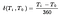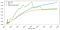# Estimating term structure using exact methods

When working on finance topics, one can rarely ignore interest rates. Interest rates are found in every corner of finance — be it a simple theory of time value of money, or pricing complex derivatives. It’s also probably the first instrument one may learn about from one’s basic business finance course (it is for me). However, as easy as it seems, there are many instruments that fall within the umbrella of interest rates, from the simplest zero-coupon bond to the complex interest rate swaps. Marveled by the beauty of interest rate, and with a hunger for a good challenge, I took up a course on “Interest Rate Models” on Coursera by Dr. Damir Filipovic of EPFL. And in this article, I’d like to share my learning experience on a very simple topic — term structure estimation using exact methods.

On a side note: When I mentioned “a good challenge”, it really is a good challenge. This course is one of few courses on the platform that were rated “advanced”, and I kid you not — I did struggle quite a bit going through the content and tests.

So why term structure for an article topic? A term structure (also known as yield curve) comes in handy for many situations. The changes in a yield curve allow one to determine the condition of an economy and thus allow governments and policymakers to develop certain strategies and plans to cope with the changing economic outlook. On a micro-level, the term structure may be used for ‘back-of-the-envelope’ calculations of prices of simple derivatives. On risk management, the term structure is necessary when marking-to-market is required. Since term structure is such an important fundamental concept, I wanted to try to explain my understanding of this particular topic as a way to consolidate my learning and share knowledge.

In an ideal situation, one may wish to obtain the market data of zero-coupon bonds (ZCBs) and money market instruments ranging from overnight to long-dated (e.g. 30 years) maturity. However, more often than not, many of these instruments do not exist. Instead, we may have various sources of market data, such as LIBOR rates, futures, and Interest rate swaps. It is essential to know how to derive the discount factor from these instruments to build up the term structure.

There are 2 general approaches to estimating the term structure — namely the exact method and a smoothing method. An exact method tries to fit the market data onto the estimated term structure. However, the attempt to fit the market data as closely as possible comes with some trade-offs, which will be covered shortly. On the contrary, a smoothing method seeks to produce an approximate shape of the curve, at the expense of market data fit.

As I’m more interested to explore this exercise from a trader’s perspective (and I highly doubt I’ll become a policymaker in my lifetime), we shall explore 2 types of exact methods — bootstrapping method and the Pseudo-inverse method.

# Understanding the Data

As mentioned earlier, the market data used in this exercise is from various sources. Before we dive into the methods, we shall quickly recap some of the basic theories.

LIBOR and Simple Spot Rates

LIBOR — short for London Inter-Bank Offer Rates — used to be the reference rate used by global banks for short-term loans. According to Investopedia, it was based on 5 major currencies and used to serve 7 maturities, from overnight, a few weeks, up to 12 months. Consider a notional value of 1 and a spot date T0, to get the discounted price (denoted P) for LIBOR (denoted L) with maturity denoted Ti, we’ll turn to the simple spot rate equation:

where delta is the fraction of the number of days difference over 360 days, which is the day convention we shall use in this exercise.The equation for delta — the period difference in years (based on 360-day convention)

Eurodollar Futures price and forward rates

An interest rate future contract is a derivative that allows both buyer and seller to secure a price on an interest-bearing asset. For simplicity, we approximate the forward rate as the futures rate, which is given by

where t is the spot date, T0 is the reset date (three months prior to maturity) and T1 is the maturity date.

Interest rate swaps

An IRS is a forward contract that allows the buyer and seller to swap a future stream of interest payments, usually fixed-to-float. The swap occurs annually, and the quotes provided are the fixed interest rates in percentage points. To get the discounted price, we shall derive it from the swap rate equation.

Through Python implementation of the above equations, we can derive a set of discounted prices, which constitute the discount curve as follows.

From this discount curve, we are able to further derive three more important curves, namely the simple spot rate curve, the yield curve, and the implied forward curve. While the discount curve looks smoothed at one glance, the imperfections from the estimations will show more prominent in those three curves. This is because these quantities are more sensitive to price changes, particularly the implied forward curve. As shown in the following image, the implied forward curve exhibits “jagged lines” at points of interpolation, highlighting the cons of the bootstrap method.Simple spot rate curve, yield curve, implied forward curve exhibit signs of discontinuities.

The bootstrapping method is much like a “Brute Force” method of constructing the discount curve and the yield curve. It does work well for many situations, but it is not an optimized solution. Filipovic presented a more elegant solution in his paper, “Exact Smooth Term-Structure Estimation” (Filipovic &Willians, 2018), hereby known as the Pseudo-inverse method.

Here I shall briefly discuss the concept without delving too much into the mathematics (A detailed breakdown of the method and implementation is available on my Github at the end of this article). First, we model the cash flow equation into a linear algebra system Cd = p, where p is the price vector at t = 0, C is the cash flow matrix of all available instruments (in our case, C is a 17 x 39 matrix), and d is the discount vector which we wish to solve.

However, this simple linear system is not sufficient. Given that we have 39 cashflow periods, but with only 17 instruments, our system is not invertible, and we can’t solve d via d= inv(C)p and that the system is under-determined. In other words, this system yields an infinite number of possible solutions of d. Intuitively, this means that our data points are too scarce that we can “plot” an infinite number of unique curves to fit these data (and unsurprisingly, this implies that our bootstrap solution is simply one of the many possible solutions).

Even though the system is not invertible, we may manipulate the system such that it is constructed with a full-ranked matrix A. With this full-ranked matrix, a Pseudo-inverse matrix A.T inv(A, A_T), where A_T is the transposed matrix of A, may be applied such that we achieve a particular solution, which also by definition, the optimized solution with minimal Euclidean norm (L2).

In this implementation, we seek to solve for a particular solution of the weighted increments vector, a vector of quantities we are able to derive via simple algebraic manipulation of the initial system. The full derivation will not be displayed in this post but is made available on the GitHub page.

With a particular solution, we can easily derive the discount vector, and from which the three rates curves.

With two methods, which is the better one? It depends, really.

If you are only concerned with getting the discount curve and the yield curve, the bootstrap method is sufficient. This method really suffered when we wish to extract an implied forward curve, which is highly sensitive to price changes. Mathematically, the Pseudo-inverse method offers an optimal solution that is smoothed (evidently from the plot below), which may be more useful in this context. So unless you need an implied forward curve, the bootstrap method is sufficient.

When it comes to implementation, a bootstrap method is more suitable on an Excel sheet, especially if the data is not huge. It may require more planning and thinking how to write functions on python such that we can manipulate the data, and it gets more complex the more diverse the sources are. The pseudo-inverse method, on the other hand, is suitable for python implementation since it is a linear system that NumPy could easily managed.

So here’s a short walkthrough on Term Structure construction and I hope you find this insightful! Please share with me your thoughts on this exercise, and also suggestions for improvement, I gladly welcome it! Thanks for reading!